• ## 基本的高通滤波器

千次阅读 2019-06-09 16:21:55
文章目录一、一个用于高通滤波的函数二、高通滤波器三、高通滤波 一、一个用于高通滤波的函数 使用函数 lpfilter 构建一个生成高通滤波器的函数：hpfilter function H = hpfilter(type, M, N, D0, n) %HPFIL...
就像低通滤波会使图像变得模糊，高通滤波通过削弱傅里叶变换的低频而保持高频相对不变，会使得图像变得更加清晰(锐化)
关于低频、高频可点击参考
文章目录一、一个用于高通滤波的函数二、高通滤波器三、高通滤波
一、一个用于高通滤波的函数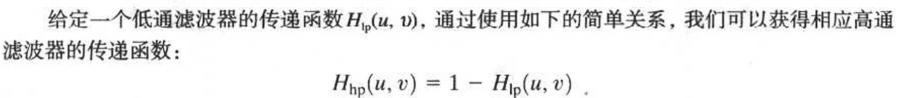因此可以借助函数 lpfilter 构建一个生成高通滤波器的函数：hpfilter
function H = hpfilter(type, M, N, D0, n)
%HPFILTER Computes frequency domain highpass filters.
%   H = HPFILTER(TYPE, M, N, D0, n) creates the transfer function of
%   a highpass filter, H, of the specified TYPE and size (M-by-N).
%   Valid values for TYPE, D0, and n are:
%
%   'ideal'    Ideal highpass filter with cutoff frequency D0.  n
%              need not be supplied. D0 must be positive.
%
%   'btw'      Butterworth highpass filter of order n, and cutoff
%              D0.  The default value for n is 1.0. D0 must be
%              positive.
%
%   'gaussian' Gaussian highpass filter with cutoff (standard
%              deviation) D0. n need not be supplied. D0 must be
%              positive.

% The transfer function Hhp of a highpass filter is 1 - Hlp,
% where Hlp is the transfer function of the corresponding lowpass
% filter.  Thus, we can use function lpfilter to generate highpass
% filters.

if nargin == 4
n = 1; % Default value of n.
end

% Generate highpass filter.
Hlp = lpfilter(type, M, N, D0, n);
H = 1 - Hlp;

二、高通滤波器
H = fftshift(hpfilter('ideal',500,500,50)); % ideal（巴特沃斯）,btw（巴特沃斯）,gaussian（高斯低通滤波器）
mesh(double(H(1:10:500,1:10:500)));
axis tight
colormap([0 0 0])
axis off
figure,imshow(H,[])

理想高通滤波器及其图像巴特沃斯滤波器及其图像高斯低通滤波器及其图像三、高通滤波
f = imread('ilpf.tif');
D0 = 0.05*PQ(1); % 使用的 D0 等于所填充图像长度的 5%
H = hpfilter('gaussian',PQ(1),PQ(2),D0); % 生成一个高通滤波器函数
g = dftfilt(f,H); % 获取滤波后的图像
imshow(f),figure,imshow(g)如图所示，图像中的边缘和其他灰度急剧转变得到了增强。
但是由于图像的平均值由 F(0,0) 给出，且迄今为止所讨论的高通滤波器偏离了傅里叶变换的原点，因此图像失去了大部分原始图像中所呈先出的灰色调。


展开全文• 下两个滤波器都是切比雪夫I型数字滤波器，不是巴特沃尔滤波器，请使用者注意！1.低通滤波器使用说明：将下列代码幅值然后以m文件保存，文件名要与函数名相同，这里函数名：lowp。function y=lowp(x,f1,f3,rp,rs,Fs) ...
本文为转载内容，原文地址为点击打开链接。下两个滤波器都是切比雪夫I型数字滤波器，不是巴特沃尔滤波器，请使用者注意！1.低通滤波器使用说明：将下列代码幅值然后以m文件保存，文件名要与函数名相同，这里函数名：lowp。function y=lowp(x,f1,f3,rp,rs,Fs)
%低通滤波
%使用注意事项：通带或阻带的截止频率的选取范围是不能超过采样率的一半
%即，f1,f3的值都要小于 Fs/2
%x:需要带通滤波的序列
% f 1：通带截止频率
% f 3：阻带截止频率
%rp：边带区衰减DB数设置
%rs：截止区衰减DB数设置
%FS：序列x的采样频率
% rp=0.1;rs=30;%通带边衰减DB值和阻带边衰减DB值
% Fs=2000;%采样率
%
wp=2*pi*f1/Fs;
ws=2*pi*f3/Fs;
% 设计切比雪夫滤波器；
[n,wn]=cheb1ord(wp/pi,ws/pi,rp,rs);
[bz1,az1]=cheby1(n,rp,wp/pi);
%查看设计滤波器的曲线
[h,w]=freqz(bz1,az1,256,Fs);
h=20*log10(abs(h));
figure;plot(w,h);title('所设计滤波器的通带曲线');grid on;
%
y=filter(bz1,az1,x);%对序列x滤波后得到的序列y
end--------------------------------------低通滤波器使用例子的代码fs=2000;
t=(1:fs)/fs;
ff1=100;
ff2=400;
x=sin(2*pi*ff1*t)+sin(2*pi*ff2*t);
figure;
subplot(211);plot(t,x);
subplot(212);hua_fft(x,fs,1);
%低通测试
% y=filter(bz1,az1,x);
y=lowp(x,300,350,0.1,20,fs);
figure;
subplot(211);plot(t,y);
subplot(212);hua_fft(y,fs,1);%hua_fft()函数是画频谱图的函数，代码在下面给出，要保存为m文件调用%这段例子还调用了我自己写的专门画频谱图的函数，也给出，不然得不出我的结果;%画信号的幅频谱和功率谱
%频谱使用matlab例子表示
function hua_fft(y,fs,style,varargin)
%当style=1,画幅值谱；当style=2,画功率谱;当style=其他的，那么花幅值谱和功率谱
%当style=1时，还可以多输入2个可选参数
%可选输入参数是用来控制需要查看的频率段的
%第一个是需要查看的频率段起点
%第二个是需要查看的频率段的终点
%其他style不具备可选输入参数，如果输入发生位置错误
nfft=2^nextpow2(length(y));%找出大于y的个数的最大的2的指数值（自动进算最佳FFT步长nfft）
%nfft=1024;%人为设置FFT的步长nfft
y=y-mean(y);%去除直流分量
y_ft=fft(y,nfft);%对y信号进行DFT，得到频率的幅值分布
y_p=y_ft.*conj(y_ft)/nfft;%conj()函数是求y函数的共轭复数，实数的共轭复数是他本身。
y_f=fs*(0:nfft/2-1)/nfft;�T变换后对应的频率的序列
% y_p=y_ft.*conj(y_ft)/nfft;%conj()函数是求y函数的共轭复数，实数的共轭复数是他本身。
if style==1
ifnargin==3
plot(y_f,2*abs(y_ft(1:nfft/2))/length(y));%matlab的帮助里画FFT的方法
%ylabel('幅值');xlabel('频率');title('信号幅值谱');
%plot(y_f,abs(y_ft(1:nfft/2)));%论坛上画FFT的方法
else
f1=varargin{1};
fn=varargin{2};
ni=round(f1 * nfft/fs+1);
na=round(fn * nfft/fs+1);
plot(y_f(ni:na),abs(y_ft(ni:na)*2/nfft));
end

elseif style==2
plot(y_f,y_p(1:nfft/2));
%ylabel('功率谱密度');xlabel('频率');title('信号功率谱');
else
subplot(211);plot(y_f,2*abs(y_ft(1:nfft/2))/length(y));
ylabel('幅值');xlabel('频率');title('信号幅值谱');
subplot(212);plot(y_f,y_p(1:nfft/2));
ylabel('功率谱密度');xlabel('频率');title('信号功率谱');
end
end下面三幅图分别是滤波前的时频图，滤波器的滤波特性曲线图和滤波后的时频图，通过图可以看出成功留下了100Hz的低频成分而把不要的高频成分去除了。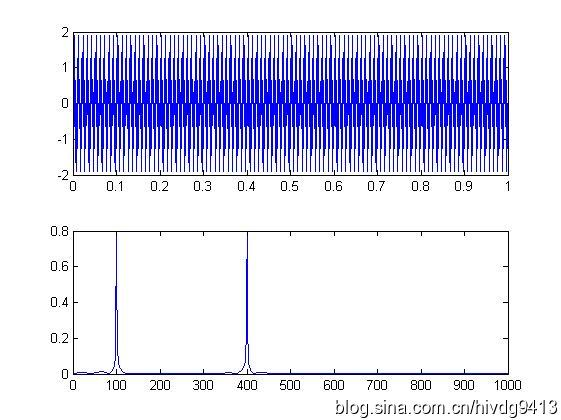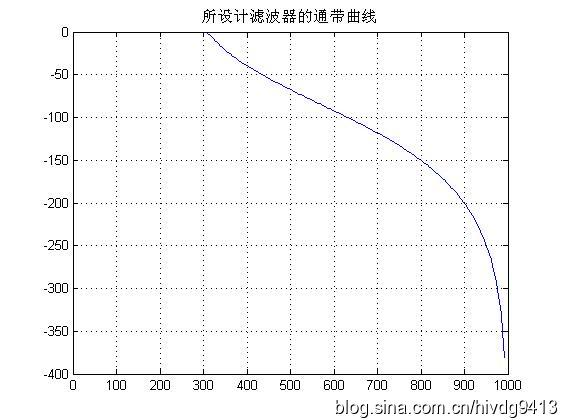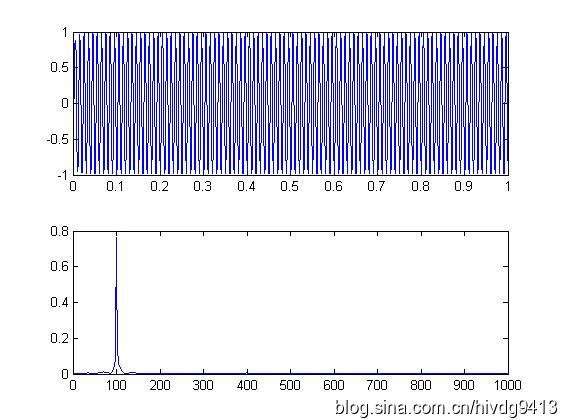2.高通滤波器function y=highp(x,f1,f3,rp,rs,Fs)
%高通滤波
%使用注意事项：通带或阻带的截止频率的选取范围是不能超过采样率的一半
%即，f1,f3的值都要小于 Fs/2
%x:需要带通滤波的序列
% f 1：通带截止频率
% f 2：阻带截止频率
%rp：边带区衰减DB数设置
%rs：截止区衰减DB数设置
%FS：序列x的采样频率
% rp=0.1;rs=30;%通带边衰减DB值和阻带边衰减DB值
% Fs=2000;%采样率
%
wp=2*pi*f1/Fs;
ws=2*pi*f3/Fs;
% 设计切比雪夫滤波器；
[n,wn]=cheb1ord(wp/pi,ws/pi,rp,rs);
[bz1,az1]=cheby1(n,rp,wp/pi,'high');

%查看设计滤波器的曲线
[h,w]=freqz(bz1,az1,256,Fs);
h=20*log10(abs(h));
figure;plot(w,h);title('所设计滤波器的通带曲线');grid on;
y=filter(bz1,az1,x);
end下面是高通滤波器的例子fs=2000;
t=(1:fs)/fs;
ff1=100;
ff2=400;
x=sin(2*pi*ff1*t)+sin(2*pi*ff2*t);
figure;
subplot(211);plot(t,x);
subplot(212);hua_fft(x,fs,1);

%------高通测试
z=highp(x,350,300,0.1,20,fs);
figure;
subplot(211);plot(t,z);
subplot(212);hua_fft(z,fs,1);下面三幅图分别是滤波前的时频图，滤波器的滤波特性曲线图和滤波后的时频图，通过图可以看出成功留下了400Hz的高频成分而把不要的低频成分100Hz去除了。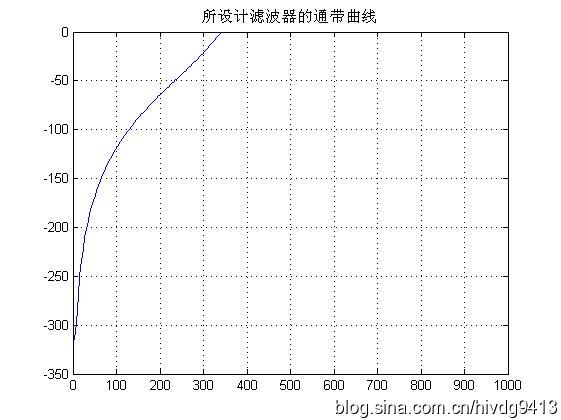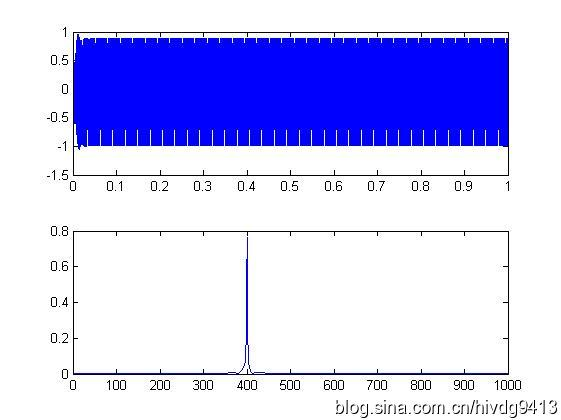3.带通滤波器function y=bandp(x,f1,f3,fsl,fsh,rp,rs,Fs)
%带通滤波
%使用注意事项：通带或阻带的截止频率与采样率的选取范围是不能超过采样率的一半
%即，f1,f3,fs1,fsh,的值小于 Fs/2
%x:需要带通滤波的序列
% f 1：通带左边界
% f 3：通带右边界
% fs1：衰减截止左边界
% fsh：衰变截止右边界
%rp：边带区衰减DB数设置
%rs：截止区衰减DB数设置
%FS：序列x的采样频率
% f1=300;f3=500;%通带截止频率上下限
% fsl=200;fsh=600;%阻带截止频率上下限
% rp=0.1;rs=30;%通带边衰减DB值和阻带边衰减DB值
% Fs=2000;%采样率
%
wp1=2*pi*f1/Fs;
wp3=2*pi*f3/Fs;
wsl=2*pi*fsl/Fs;
wsh=2*pi*fsh/Fs;
wp=[wp1 wp3];
ws=[wsl wsh];
%
% 设计切比雪夫滤波器；
[n,wn]=cheb1ord(ws/pi,wp/pi,rp,rs);
[bz1,az1]=cheby1(n,rp,wp/pi);
%查看设计滤波器的曲线
[h,w]=freqz(bz1,az1,256,Fs);
h=20*log10(abs(h));
figure;plot(w,h);title('所设计滤波器的通带曲线');grid on;
y=filter(bz1,az1,x);
end带通滤波器使用例子%--------------
%带通滤波器测试程序
fs=2000;
t=(1:fs)/fs;
ff1=100;
ff2=400;
ff3=700;
x=sin(2*pi*ff1*t)+sin(2*pi*ff2*t)+sin(2*pi*ff3*t);
figure;
subplot(211);plot(t,x);
subplot(212);hua_fft(x,fs,1);
% y=filter(bz1,az1,x);
y=bandp(x,300,500,200,600,0.1,30,fs);
figure;
subplot(211);plot(t,y);
subplot(212);hua_fft(y,fs,1);%调用到的hua_fft()函数代码如下function hua_fft(y,fs,style,varargin)
%当style=1,画幅值谱；当style=2,画功率谱;当style=其他的，那么花幅值谱和功率谱
%当style=1时，还可以多输入2个可选参数
%可选输入参数是用来控制需要查看的频率段的
%第一个是需要查看的频率段起点
%第二个是需要查看的频率段的终点
%其他style不具备可选输入参数，如果输入发生位置错误
nfft=2^nextpow2(length(y));%找出大于y的个数的最大的2的指数值（自动进算最佳FFT步长nfft）
%nfft=1024;%人为设置FFT的步长nfft
y=y-mean(y);%去除直流分量
y_ft=fft(y,nfft);%对y信号进行DFT，得到频率的幅值分布
y_p=y_ft.*conj(y_ft)/nfft;%conj()函数是求y函数的共轭复数，实数的共轭复数是他本身。
y_f=fs*(0:nfft/2-1)/nfft;�T变换后对应的频率的序列
% y_p=y_ft.*conj(y_ft)/nfft;%conj()函数是求y函数的共轭复数，实数的共轭复数是他本身。
if style==1
ifnargin==3
plot(y_f,2*abs(y_ft(1:nfft/2))/length(y));%matlab的帮助里画FFT的方法
%ylabel('幅值');xlabel('频率');title('信号幅值谱');
%plot(y_f,abs(y_ft(1:nfft/2)));%论坛上画FFT的方法
else
f1=varargin{1};
fn=varargin{2};
ni=round(f1 * nfft/fs+1);
na=round(fn * nfft/fs+1);
plot(y_f(ni:na),abs(y_ft(ni:na)*2/nfft));
end

elseif style==2
plot(y_f,y_p(1:nfft/2));
%ylabel('功率谱密度');xlabel('频率');title('信号功率谱');
else
subplot(211);plot(y_f,2*abs(y_ft(1:nfft/2))/length(y));
ylabel('幅值');xlabel('频率');title('信号幅值谱');
subplot(212);plot(y_f,y_p(1:nfft/2));
ylabel('功率谱密度');xlabel('频率');title('信号功率谱');
end
end运行结果如下图，第一幅是滤波前测试信号的时频图，第二幅是滤波器的滤波曲线图，第三幅是经滤波后的测试信号时频图。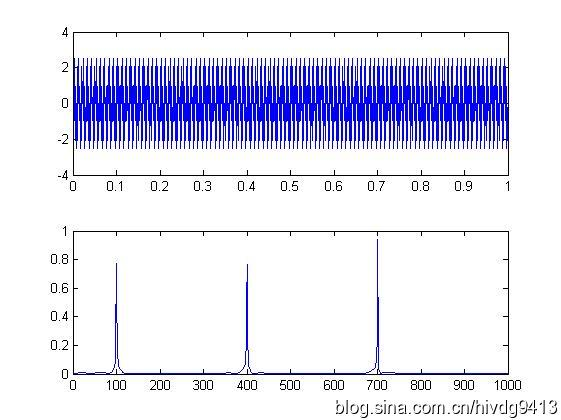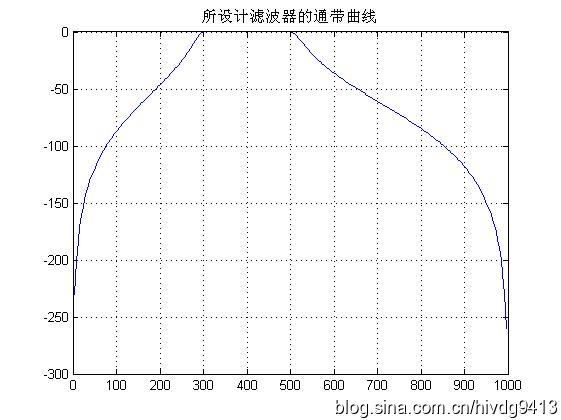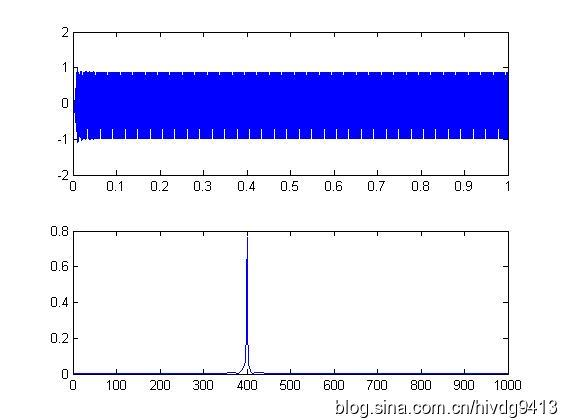4.带阻滤波器function y=bands(x,f1,f3,fsl,fsh,rp,rs,Fs)
%带阻滤波
%使用注意事项：通带或阻带的截止频率与采样率的选取范围是不能超过采样率的一半
%即，f1,f3,fs1,fsh,的值小于 Fs/2
%x:需要带通滤波的序列
% f 1：通带左边界
% f 3：通带右边界
% fs1：衰减截止左边界
% fsh：衰变截止右边界
%rp：边带区衰减DB数设置
%rs：截止区衰减DB数设置
%FS：序列x的采样频率
% f1=300;f3=500;%通带截止频率上下限
% fsl=200;fsh=600;%阻带截止频率上下限
% rp=0.1;rs=30;%通带边衰减DB值和阻带边衰减DB值
% Fs=2000;%采样率
%
wp1=2*pi*f1/Fs;
wp3=2*pi*f3/Fs;
wsl=2*pi*fsl/Fs;
wsh=2*pi*fsh/Fs;
wp=[wp1 wp3];
ws=[wsl wsh];
%
% 设计切比雪夫滤波器；
[n,wn]=cheb1ord(ws/pi,wp/pi,rp,rs);
[bz1,az1]=cheby1(n,rp,wp/pi,'stop');
%查看设计滤波器的曲线
[h,w]=freqz(bz1,az1,256,Fs);
h=20*log10(abs(h));
figure;plot(w,h);title('所设计滤波器的通带曲线');grid on;
y=filter(bz1,az1,x);
end使用例子%带阻滤波器测试
fs=1000;
t=(1:fs)/fs;
y=sin(2*pi*100*t)+sin(2*pi*150*t)+sin(2*pi*200*t);
figure;hua_fft(y,fs,1);
z=bands(y,110,190,140,160,0.1,30,fs);
figure;hua_fft(z,fs,1);运行结果如下图，第一幅是滤波前测试信号的频谱图，第二幅是滤波器的滤波曲线图，第三幅是经滤波后的测试信号频谱图。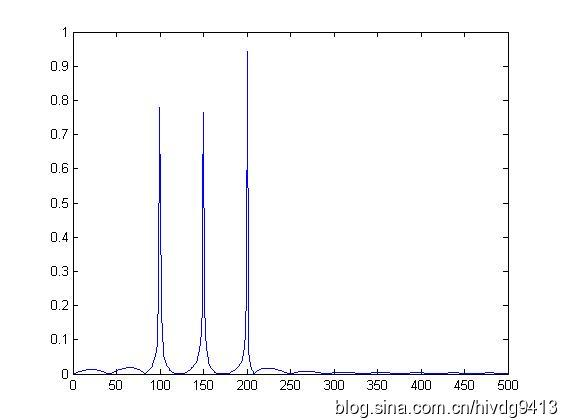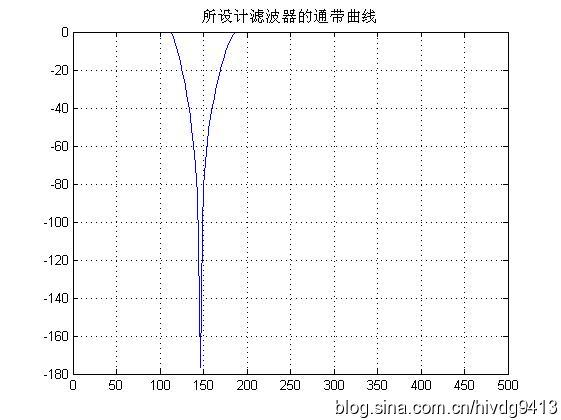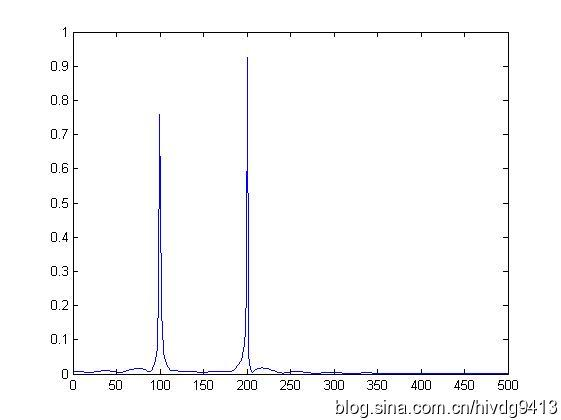展开全文低通滤波器 带通滤波器 带阻滤波器 matlab
• 傅立叶变换,拉普拉斯变换和Z变换 对于信号分析而言,傅立叶变换是必不可少的,我们都知道傅立叶变换是把系统从时域变换到频域进行分析,那么拉普拉斯变换和Z变换是干什么的?简单的来说,由于傅里叶变换的收敛有一个...
傅立叶变换,拉普拉斯变换和Z变换

对于信号分析而言,傅立叶变换是必不可少的,我们都知道傅立叶变换是把系统从时域变换到频域进行分析,那么拉普拉斯变换和Z变换是干什么的?简单的来说,由于傅里叶变换的收敛有一个狄利克雷条件，要求信号绝对可积/绝对可和。对于那些不符合狄利克雷条件的信号该怎么办呢,我们将频域的概念扩展到复频域.首先要说明的是傅立叶变换大致有两种,连续时间的傅立叶变换(CTFT)和离散的傅立叶变换(DTFT).而对于CTFT而言,拉普拉斯变换就是将连续时间系统的傅立叶变换扩展了;而对于DTFT而言,Z变换就是将离散时间系统的傅立叶变换扩展了.知乎上有一个很好的对三种变换的解释:傅立叶变换、拉普拉斯变换、Z变换的联系

RC一阶低通滤波器的算法推导

一阶的RC电路如下: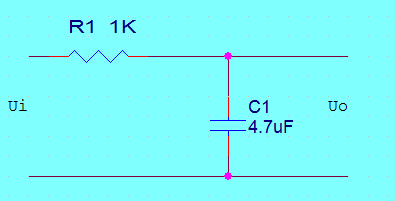这里直接给出其s域的传递函数:
VoutVin=1RCs+1,(s=jω)$\frac{{\mathbf{V}}_{\mathbf{o}\mathbf{u}\mathbf{t}}}{{\mathbf{V}}_{\mathbf{i}\mathbf{n}}}=\frac{1}{RCs+1},\left(s=j\omega \right)$
\frac{\mathbf{V_{out}}}{\mathbf{V_{in}}}=\frac{1}{RCs+1},(s=j\omega)

对其进行z变换(一阶后差分):
s=1−z−1T,T表示采样周期$s=\frac{1-{z}^{-1}}{T},T表示采样周期$$s=\frac{1-z^{-1}}{T},T表示采样周期$
则传递函数变为:
Y(z)X(z)=TRC(1−Z−1)+T$\frac{Y\left(z\right)}{X\left(z\right)}=\frac{T}{RC\left(1-{Z}^{-1}\right)+T}$
\frac{Y(z)}{X(z)}=\frac{T}{RC(1-Z^{-1})+T}

又因为Y(z)=Y(n)z−n,且Y(n−1)Y(n)=z−1$Y\left(z\right)=Y\left(n\right){z}^{-n},且\frac{Y\left(n-1\right)}{Y\left(n\right)}={z}^{-1}$$Y(z)=Y(n)z^{-n},且\frac{Y(n-1)}{Y(n)}=z^{-1}$,X(z)=X(n)z−n,且X(n−1)X(n)=z−1$X\left(z\right)=X\left(n\right){z}^{-n},且\frac{X\left(n-1\right)}{X\left(n\right)}={z}^{-1}$$X(z)=X(n)z^{-n},且\frac{X(n-1)}{X(n)}=z^{-1}$,代入到上式的传递函数得:
Y(n)=TT+RCX(n)+RCT+RCY(n−1)$Y\left(n\right)=\frac{T}{T+RC}X\left(n\right)+\frac{RC}{T+RC}Y\left(n-1\right)$
Y(n)=\frac{T}{T+RC}X(n)+\frac{RC}{T+RC}Y(n-1)

其中:
X(n):本次采样值$X\left(n\right):本次采样值$$X(n):本次采样值$
Y(n−1):上次滤波值$Y\left(n-1\right):上次滤波值$$Y(n-1):上次滤波值$
令a=TT+RC≈TRC=ωT=2πfT$a=\frac{T}{T+RC}\approx \frac{T}{RC}=\omega T=2\pi fT$$a=\frac{T}{T+RC}\approx \frac{T}{RC}=\omega T=2\pi fT$
则滤波公式为:
Y(n)=a∗X(n)+(1−a)∗Y(n−1)$Y\left(n\right)=a\ast X\left(n\right)+\left(1-a\right)\ast Y\left(n-1\right)$Y(n)=a*X(n)+(1-a)*Y(n-1)
这与px4代码的lib库中低通滤波是一样的:

float BlockLowPass::update(float input)
{
if (!PX4_ISFINITE(getState())) {
setState(input);
}

float b = 2 * float(M_PI) * getFCut() * getDt();
float a = b / (1 + b);
setState(a * input + (1 - a)*getState());//input:本次采样值 getState():上次滤波值
return getState();
}

一阶RC高通滤波器

RC高通滤波器原理图如下,它和低通相反,电阻两端的电压作为输出,则其s域的传递函数为:
VoutVin=RCsRCs+1$\frac{{\mathbf{V}}_{\mathbf{o}\mathbf{u}\mathbf{t}}}{{\mathbf{V}}_{\mathbf{i}\mathbf{n}}}=\frac{RCs}{RCs+1}$\frac{\mathbf{V_{out}}}{\mathbf{V_{in}}}=\frac{RCs}{RCs+1}
z$z$$z$变换(一阶后向差分):
s=1−z−1T$s=\frac{1-{z}^{-1}}{T}$s=\frac{1-z^{-1}}{T}
得到z$z$$z$域的传递函数为:
Y(z)X(z)=RC(1−z−1)RC(1−z−1)+T$\frac{Y\left(z\right)}{X\left(z\right)}=\frac{RC\left(1-{z}^{-1}\right)}{RC\left(1-{z}^{-1}\right)+T}$\frac{Y(z)}{X(z)}=\frac{RC(1-z^{-1})}{RC(1-z^{-1})+T}
同样的,Y(z)=Y(n)z−n,且Y(n−1)Y(n)=z−1$Y\left(z\right)=Y\left(n\right){z}^{-n},且\frac{Y\left(n-1\right)}{Y\left(n\right)}={z}^{-1}$$Y(z)=Y(n)z^{-n},且\frac{Y(n-1)}{Y(n)}=z^{-1}$,X(z)=X(n)z−n,且X(n−1)X(n)=z−1$X\left(z\right)=X\left(n\right){z}^{-n},且\frac{X\left(n-1\right)}{X\left(n\right)}={z}^{-1}$$X(z)=X(n)z^{-n},且\frac{X(n-1)}{X(n)}=z^{-1}$,则有:
Y(n)=RCRC+T(X(n)−X(n−1)+Y(n−1))$Y\left(n\right)=\frac{RC}{RC+T}\left(X\left(n\right)-X\left(n-1\right)+Y\left(n-1\right)\right)$Y(n)=\frac{RC}{RC+T}(X(n)-X(n-1)+Y(n-1))
其中:
X(n):本次采样值$X\left(n\right):本次采样值$$X(n):本次采样值$
X(n−1):上次采样值$X\left(n-1\right):上次采样值$$X(n-1):上次采样值$
Y(n−1):上次滤波值$Y\left(n-1\right):上次滤波值$$Y(n-1):上次滤波值$
我们令令b=TT+RC≈TRC=ωT=2πfT$b=\frac{T}{T+RC}\approx \frac{T}{RC}=\omega T=2\pi fT$$b=\frac{T}{T+RC}\approx \frac{T}{RC}=\omega T=2\pi fT$,a=11+b$a=\frac{1}{1+b}$$a=\frac{1}{1+b}$
则高通滤波的算法公式为:
Y(n)=b∗(X(n)−X(n−1)+Y(n−1))$Y\left(n\right)=b\ast \left(X\left(n\right)-X\left(n-1\right)+Y\left(n-1\right)\right)$
Y(n)=b*(X(n)-X(n-1)+Y(n-1))

这与px4中的高通滤波是一样的:


float BlockHighPass::update(float input)
{
float b = 2 * float(M_PI) * getFCut() * getDt();
float a = 1 / (1 + b);
setY(a * (getY() + input - getU()));//getY():上次滤波器输出值;getU():上次滤波器输入值
setU(input);
return getY();
}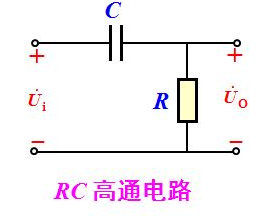总结

关于低通滤波和高通滤波,最关键的是学到了三类变换的关系以及离散化的方法,留下各位大佬的博客链接在此:
【滤波器学习笔记】一阶RC低通滤波
傅立叶变换、拉普拉斯变换、Z变换的联系
基础电路—RC组成的低通、高通滤波器
双线性变换
z变换
展开全文• ## 高通滤波器分析

万次阅读 2018-05-29 21:06:52
转载自玩转嵌入式公众号--点击打开链接曾经为了学高通滤波器的原理，还亲自用示波器测过信号的波形，自从做了那个实验，从此对高通滤波器有了更深一步的理解。要制作高通滤波器或者低通滤波器，必须要先明白他们呢的...
转载自玩转嵌入式公众号--点击打开链接曾经为了学高通滤波器的原理，还亲自用示波器测过信号的波形，自从做了那个实验，从此对高通滤波器有了更深一步的理解。要制作高通滤波器或者低通滤波器，必须要先明白他们呢的含义，他们的特性如下：高通滤波：高频信号可以通过，而低频信号不能通过。低通滤波：低频信号可以通过，而高频信号不能通过。高通滤波器的制作如下图所示为高通滤波电路的原理图，它的原理很简单，一个电容和一个电阻就构成了高通滤波电路，它只运用了一个原理：就是电容的“隔直通交”，即高频电流能通过电容，电容对高频电来说就是短路；而由于电容的“隔直通交”，对于低频信号而言，电容就是断路的，低频信号不能通过。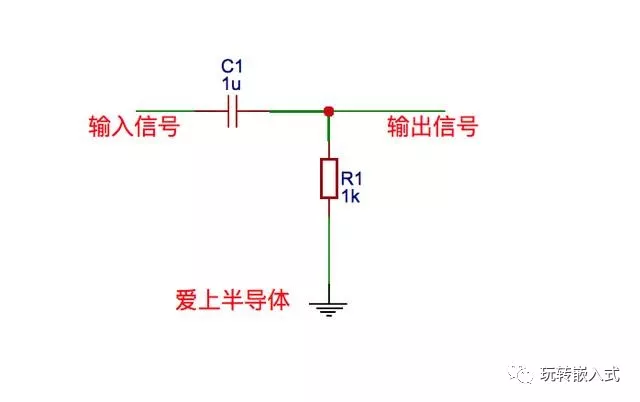现在虽然知道了高频信号能通过，那么这时候就又有了疑问，就是这个高频信号最低是多少呢，低于这个值信号就不能通过了。这就涉及到RC公式了，在模电中，有一个名词叫做截止频率，这现在的情况而言就是，它的最低频率，低于截止频率的信号不能通过，截止频率的公式为：f=1/（2πRC）,假如上述电阻为10K，电容为0.78uf，则截止频率为;f=1/（2πRC）=20Hz.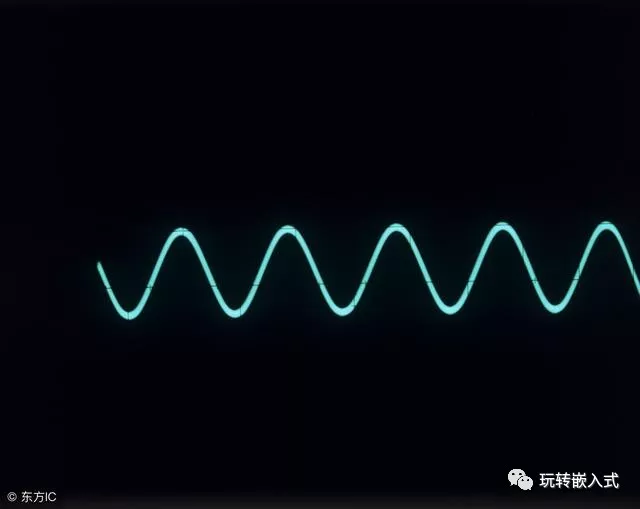低通滤波器的制作如果高通滤波器学会了，低通滤波电路自然就迎刃而解了，下面对低通滤波电路做一个简短的分析，对于高频信号，电容相当于对地短路，所以高频信号就到此为止了，低频信号由于不能通过电容，这时候会作为输出信号传输给我们。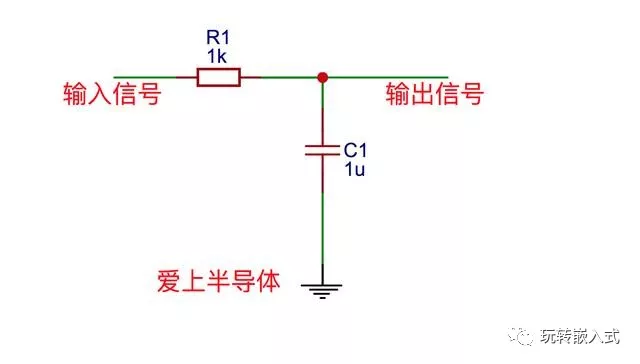同样，低通滤波信号也有一个截止频率，这个截止频率是指的能通过的最高频的信号，当再高于这个信号时，会对地短路，它的公式和高通滤波是一样的即：f=1/（2πRC）。假如上述电阻为1K，电容为0.13uf，则截止频率为f=1/（2πRC）=1224Hz，也就是只有低于1224Hz的信号能通过。
展开全文• Vo/Vi=电阻R/电路的总阻抗； 推导过程如下： ω=1/RC的时候取值0.707，此时就是截至频率也就是3db频率。
• 高通滤波器 允许高于截止频率的信号通过。是一种让某一频率以上的信号分量通过，而对该频率以下的信号分量大大抑制的电容、电感与电阻等器件的组合装置。其特性在时域及频域中可分别用冲激响应及频率响应描述。 ...
• 版权声明：<–本博客所有内容均为自己在学习工作中的总结、摘录等-- --转载请注明出处-- --如有侵权请联系删除–>...%GULS 高斯低通滤波器,D0为截至频率的（相当于设置在傅里叶谱图的半径值）...
• import cv2 import numpy as np from scipy import ndimage #高通滤波器，这些滤波器中所有的值加起来为0 kernel_3x3 = np.array([[-1,-1,-1], [-1, 8,-1], [-1,-1,-1]]) kernel_5x5 = ...opencv
• 一、引出 在一些信号电路中通常会存在一些我们不需要的噪声，比如高频噪声。这个时候就需要滤波器来处理他们了。 ...意味着，高通RC滤波器会对低于141Hz的信号起抑制作用。低通滤波器会对高于...
• ## 理想高通滤波器

万次阅读 2016-07-07 22:17:12
高通滤波器 首先，对一副图像进行如下二维傅里叶变换。 我们将u=0和v=0带上式，我们可以得到如下式子。 根据上式，可以到F(0,0)的值是非常大的。这里，我们将 F(0,0)称为直流分量，直流分量比其他的成分要...
• ## 高通滤波器

千次阅读 2018-06-12 11:59:12
• 如果大家要设计高通滤波器，大家可以和低通滤波器一起考虑~~~~ ———————————————————————————————— example1: { in there we can see: R=1/C } finally ...设计
• 一阶RC高通滤波器的matlab仿真和C语言实现，有图有代码，欢迎拍砖数字滤波器 matlab simulink
• 简单记录下在matlab上如何设计出模拟的高通滤波器，包括：巴特沃斯滤波器、切比雪夫I型滤波器、切比雪夫II型滤波器、椭圆型滤波器。 %设计高通滤波器 %巴特沃斯、切比雪夫I型、切比雪夫II型、椭圆型滤波器 ...
•matlab
• # -- coding: utf-8 -- import cv2 import cv2 import numpy as np from scipy import ndimage#这个是滤波器使用的模板矩阵 kernel_3x3 = np.array([[-1, -1, -1], [-1, 8, -1], [-python
• ## 高斯高通滤波器

万次阅读 2016-04-11 21:56:21
3.根据u=rows,h=colums计算D和H，然后用H对中心对称的图像滤波也就是H乘以变换后的图像 4.傅里叶逆变换ifft2 5.中心对称逆变换ifftshift(它与fftshift互逆) 6.记得图像要转变uint8我也不知道为什么（让我拖延一下） ...matlab 图像处理 滤波
• I1=imread('原图.jpg');figure(1);imshow(I1);title('原图');m=double(I1);f=fft2(m);f=fftshift(f);[N1,N2]=size(f);n1=round(N1/2);n2=round(N2/2);...for i=1:N1 for j=1:N2 d=sqrt((i-n1)^2+(j-n2)...
• 1、高斯高通滤波器 传递函数： 高斯低通滤波器 传递函数： D0指截止频率。 2、巴特沃斯高通滤波器 传递函数： 巴特沃斯低通滤波器 传递函数： 描述：在公式中，D(u,v)代表频域当中，...matlab 图像处理
• ## 一阶高通滤波器

千次阅读 2017-06-21 08:08:10
RC电路如下： 推导过程如下： 参考博文：http://blog.csdn.net/luoshi006/article/details/51459884
• 分别用压控电压源和无限增益多路反馈二种方法设计电路； 截止频率fc=100Hz 增益Av=5
• 将多重反馈型低通滤波器上的电阻和电容的位置对换后就成为了高通滤波器，其形式如图1 所示。 图1: 多重反馈型低通滤波器 列写电路方程如下： 化简后可得到： 当C1 = C2 = C3 = C 时，上面的式子可以化简为...
• fc=0.3KHz，δ=0.8dB，fr=0.2KHz，At=20dB，T=1ms；设计一个切比雪夫高通滤波器，观察其通带损耗和阻带衰减是否满足要求python图像处理 频率域滤波